In contourplot , the option contours=n means that if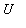and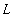are the maximum and minimum function values on the grid of points that Maple uses for the plot, the contour values are at intervals of length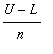. Sinceandthemselves will generally not correspond to curves on the plot, there will actually be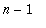contours. In contourplot3d or plot3d(..., style=contour) , on the other hand, Maple takes the contour values to be integer multiples of a number that is 1, 2 or 5 times a power of 10, using the smallest number of this form that will produce at least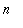contour values. This was also the behaviour of contourplot prior to Release 4.

On the other hand, you can use contours= [ a list of values ] , which gives you full control of the values that are plotted.

Examples:

> with(plots,contourplot3d, contourplot):

> contourplot3d(x,x=0..1,y=0..1,contours=4);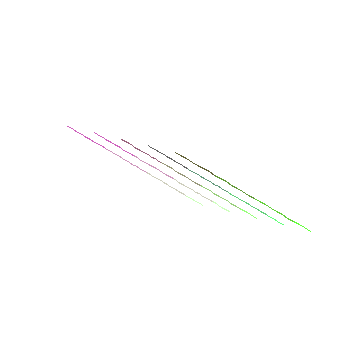> contourplot(x,x=0..1,y=0..1,contours=4);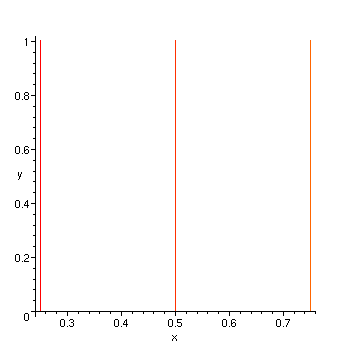> contourplot(x,x=0..1,y=0..1,contours=[.2, .4, .6, .8]);